Courses

DC Pandey Solutions: Reflection of Light Notes | Study DC Pandey Solutions for NEET Physics - JEE

JEE: DC Pandey Solutions: Reflection of Light Notes | Study DC Pandey Solutions for NEET Physics - JEE

The document DC Pandey Solutions: Reflection of Light Notes | Study DC Pandey Solutions for NEET Physics - JEE is a part of the JEE Course DC Pandey Solutions for NEET Physics.
All you need of JEE at this link: JEE

Introductory Exercise 27.1

Ques 1: Show that the unit of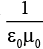is m/s.
Sol: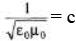= Speed of light in vacuum.

Ques 2: The magnetic field in a plane electromagnetic wave is given by (SI units)
By = (2 × 10-7 T) sin [500 x + 1.5 × 1011 t]
(a) What is the wavelength and frequency of the wave?
(b) Write an expression for the electric field vector.
Ans: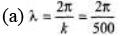= 0.0125 m= 1.25 cm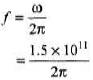= 2.39 × 1010Hz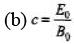E0 = cB0
= (3 × 108)(2 × 10-7) = 60 V/m
kx and ωt have same sign. Therefore, electromagnetic wave is travelling along negative x direction.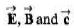are in mutually perpendicular direct ions. Hence oscillations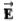of are along z-direction.

Introductory Exercise 27.2

Ques 1: Prove that for any value of angle i, rays 1 and 2 are parallel.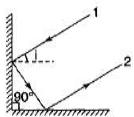Sol: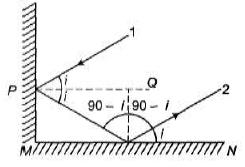PQ and MN are mutually parallel. Rays 1 and 2 are making equal angles (= ∠i) from PQ and MN. So, they are mutually parallel.

Ques 2: A man approaches a vertical plane mirror at speed of 2 m/s. At what rate does he approach his image?
Sol: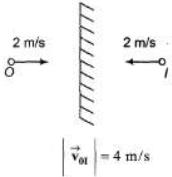Ques 3: A pole of height 4 m is kept in front of a vertical plane mirror of length 2 m. The lower end of the mirror is at a height of 6 m from the ground. The horizontal distance between the mirror and the pole is 2 m. Up to what minimum and maximum heights a man can see the image of top of the pole at a horizontal distance of 4 m (from the mirror) standing on the same horizontal line which is passing through the pole and the horizontal point below the mirror?
Sol: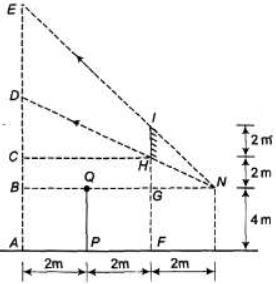PQ = Pole
MN = Image of pole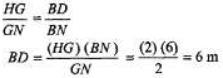Further,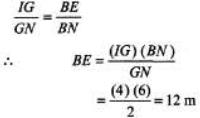Maximum height required = AE = BE+ AB = 16 m

Introductory Exercise 27.3

Ques 1: Assume that a certain spherical mirror has a focal length of- 10.0 cm. Locate and describe the image for object distances of
(a) 25.0 cm (b) 10.0 cm (c) 5.0 cm.
Sol:
Apply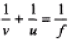(a) f = -10 cm, u = - 25 cm Solving we get, v = -16.7cm
Since, v is negative, image is in front of mirror. So it is real. Similarly, we can solve for other parts.

Ques 2: A ball is dropped from rest 3.0 m directly above the vertex of a concave mirror that has a radius of 1.0 m and lies in a horizontal plane.
(a) Describe the motion of ball's image in the mirror.
(b) At what time do the ball and its image coincide?
Sol:
(a) From O to F, image is real.
From F to P, image is virtual.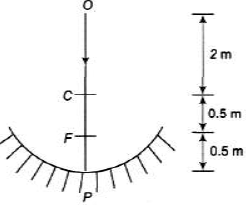At O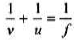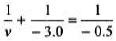v = - 0.6 m

(b) When object is at C and P, image coincides with object. Using,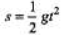or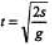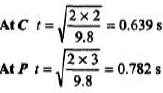Ques 3: A spherical mirror is to be used to form on a screen 5.0 m from the object an image five times the size of the object.
(a) Describe the type of mirror required.
(b) Where should the mirror be positioned relative to the object?
Sol:
(a) Image has to be taken on a screen. So it should be real. Hence mirror should be concave.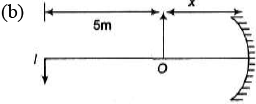Image is 5 times magnified. Hence, | v | = 5 | u |
or
(5 + x) = 5x
Solving we get, x = 1.25 cm
Now using,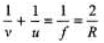We have,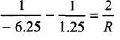Solving we get, R = - 2.08 m

Ques 4: Figure shows two rays P and Q being reflected by a mirror and going as F and Q'. State which type of mirror is this?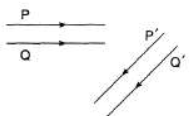Sol:
Reflected rays are neither converging nor diverging. Hence, mirror is plane.

Ques 5: The following table shows object distance, object size, and mirror focal length (all in centimeters) for various situations. Use ray tracing to determine the image size and location for each case.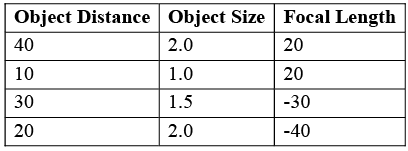Sol:
Let us draw the ray diagram for the first part.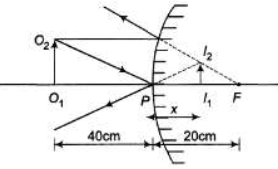In ΔO1O2P and ΔP I1I2 we have,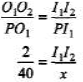or
x = (20)(I1,I2) ...(i)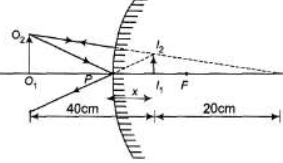In ΔO1O2C and l1I2 C we have,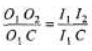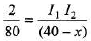or (40 - x) = 40(I1I2) ....(ii)
Solving Eqs. (i) and (ii) we get,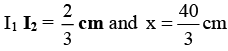Ques 6: Complete the following table for spherical mirrors. All distances are in centrimeters.

 Mirror Type Radius Focal Length Object Distance Image Distance Lateral Magnification Real/Virtual Image -25 40 20 -30 +15 +30 20 -2.0 +20 -40 -10 +2.0

Sol: Negative focal length means concave mirror and positive focal length means convex mirror.
Apply,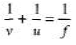And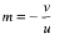Further, if v is negative then image is in front of mirror. So, it is real and inverted.
R = 2f

The document DC Pandey Solutions: Reflection of Light Notes | Study DC Pandey Solutions for NEET Physics - JEE is a part of the JEE Course DC Pandey Solutions for NEET Physics.
All you need of JEE at this link: JEEUse Code STAYHOME200 and get INR 200 additional OFF Use Coupon Code

Top Courses for JEE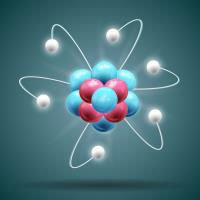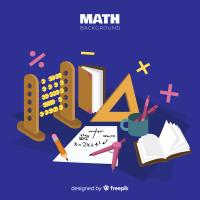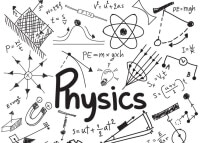DC Pandey Solutions for NEET Physics

132 docs

Top Courses for JEETrack your progress, build streaks, highlight & save important lessons and more!

,

,

,

,

,

,

,

,

,

,

,

,

,

,

,

,

,

,

,

,

,

;